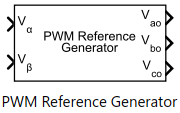# Space Vector Generator

Generate space vector modulation signals

• Library:
• Motor Control Blockset / Controls / Math Transforms

•## Description

The Space Vector Generator block generates space vector modulation voltage signals from stator reference voltages described by α-β voltage components.

The block uses a combination of inverse Clarke transformation and Space Vector Pulse Width Modulation (SVPWM) to compute modulated DC output voltages.

This figure shows the input reference voltages and the genearted space vector modulation voltage signals:## Ports

### Input

expand all

Stator reference voltage component along α-axis of the αβ reference frame.

Data Types: `single` | `double` | `fixed point`

Stator reference voltage component along β-axis of the αβ reference frame.

Data Types: `single` | `double` | `fixed point`

### Output

expand all

Stator reference voltage component along a-axis of the abc reference frame.

Data Types: `single` | `double` | `fixed point`

Stator reference voltage component along b-axis of the abc reference frame.

Data Types: `single` | `double` | `fixed point`

Stator reference voltage component along c-axis of the abc reference frame.

Data Types: `single` | `double` | `fixed point`

Get ebook